Select Page

# CBSE Application of Derivatives Maths 12 Science Answers for MCQ in English

CBSE Application of Derivatives Maths 12 Science Answers for MCQ in English to enable students to get Answers in a narrative video format for the specific question.

Expert Teacher provides CBSE Application of Derivatives Maths 12 Science Answers for MCQ through Video Answers in English language. This video solution will be useful for students to understand how to write an answer in exam in order to score more marks. This teacher uses a narrative style for a question from Application of Derivatives not only to explain the proper method of answering question, but deriving right answer too.

Please find the question below and view the Answer in a narrative video format.

Question:

## Similar Questions from CBSE, 12th Science, Maths, Application of Derivatives

Question 1 : The line y=mx+1 is a tangent to the curve if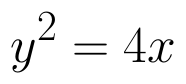the value of m is ________. (View Answer Video)

Question 2 : If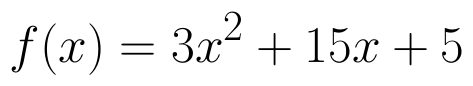, then the approximate value of f (3.02) is  (View Answer Video)

Question 3 : The volume of a cube is increasing at the rate of 9 cubic centimetres per second. How fast is the surface area increasing when the length of an edge is 10 centimetres? (View Answer Video)

Question 4 : The maximum value of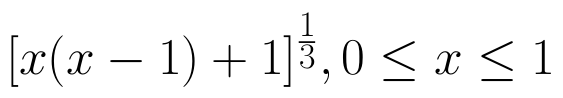is, (View Answer Video)

Question 5 : A balloon, which always remains spherical has a variable radius. Find the rate at which its volume is increasing with the radius when the later is 10 cm.  (View Answer Video)

### Differential Equations

Question 1 : Determine degree of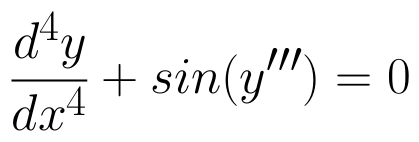(View Answer Video)

Question 2 : Write the degree of the differential equation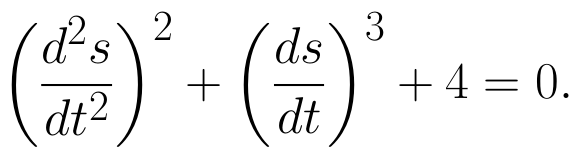(View Answer Video)

Question 3 : Solve the differential equation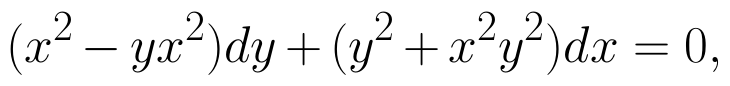given that y = 1 when x = 1. (View Answer Video)

Question 4 : Show that the solution of differential equation :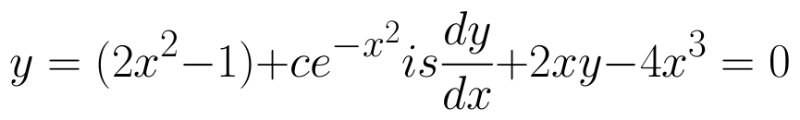(View Answer Video)

Question 5 : Write the degree of the differential equation :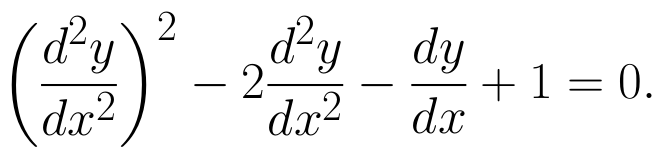(View Answer Video)

### Three Dimensional Geometry

Question 1 : Find the distance between the point (5, 4, -6) and its image in xy-plane. (View Answer Video)

Question 2 : Find the distance between the point (-1, -5, -10) and the point of intersection of line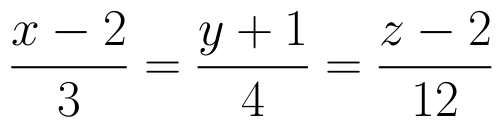and plane
Question 3 : Find the vector equation of the line passing through the point (1, 2, 3) and parallel to the planes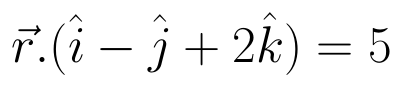and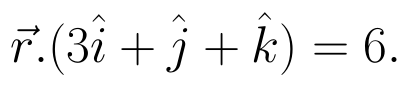(View Answer Video)
Question 4 : Find the vector equation of the plane which is at a distance of 5 units from the origin and normal to the plane is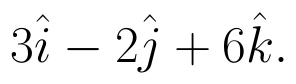(View Answer Video)
Question 5 : Find the vector equation of the plane which contains the line of intersection of the planes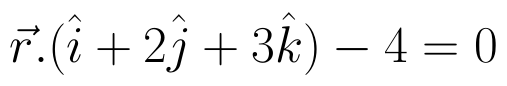and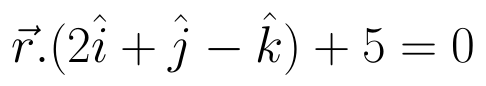and which is perpendicular to the plane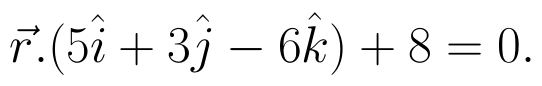(View Answer Video)